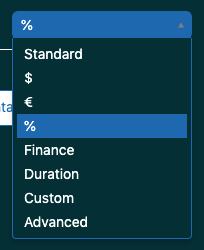Community Moderator

Hi Nick,

You can get the % of chats answered within 3 minutes this way:

## Step 1: Create a calculated metric

The first step is to create a calculated metrics that give you the number of chats with a first reply time of less than 3 minutes.

1. Go to Calculations > Standard calculated metric.
2. Give the formula a name and past in the following:
`IF (VALUE(Chat first reply time (sec))<180) THEN [Chat ID]ENDIF`
3. Save the metric.

If you want to do it for engagement first reply time, switch out Chat first reply time (sec) with Engagement first reply time (sec) in the formula.

## Step 2: Select metrics

The next step is to select your metrics.

1. First, select the newly created metric. Set the aggregator to D_COUNT.
2. Secondly, select the metric Chats.

## Step 3: Select attributes

Here you select the attributes that you want the metric(s) to be dived by. Put them in Rows. For Chat first reply time (sec) I would select Chat started - Date and for Engagement first reply time (sec) I would select Engagement - Date.

## Step 4: Display as % of total

The last step is to display the percentage of chats answered within 3 minutes out of the total amount of chats.

1. Go to Result manipulation > Result metric calculation.
2. Add a new metric, give it a new and put in the following (remember to switch out the "name of your metric" with the actual name of your metric):
`D_COUNT(name of your metric)/COUNT(Chats)`
3. Select Clear used metrics if you only what the percentage to be shown.
5. Go to Chart configuration > Display format.
6. Select % from the dropdown.7. You should now have a table that displays the percentage of chats answered within 3 minutes out of the total number of chats.

I hope this helps :)

#helpsome regards,
Marie-Cathrine Sørensen
Developer @ helphouse.io

Worked like a charm! Thank you Marie-Cathrine!